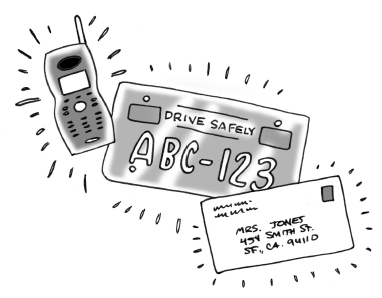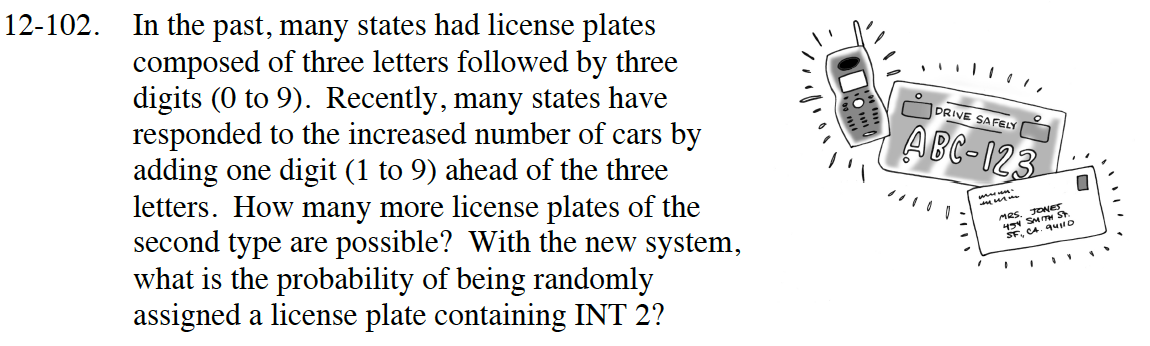### Home > INT2 > Chapter 12 > Lesson 12.2.3 > Problem12-102

12-102.
1.In the past, many states had license plates composed of three letters followed by three digits (0 to 9). Recently, many states have responded to the increased number of cars by adding one digit (1 to 9) ahead of the three letters. How many more license plates of the second type are possible? With the new system, what is the probability of beingrandomly assigned a license plate containing INT 2? Homework Help ✎Since repetition is allowed, the total number of license plates of the first type is
26 · 26· 26 · 10· 10 · 10 = 17,576,000.

The total number of license plates of the second type is
9 · 26 · 26 · 26 · 10 · 10 · 10 = 158,184,000.

Find the difference.

Find the number of license plates containing INT2.

9 · 1 · 1 · 1 · 1 · 10 · 10 =

$P(INT2) = \frac{900}{158,184,000} = ?$